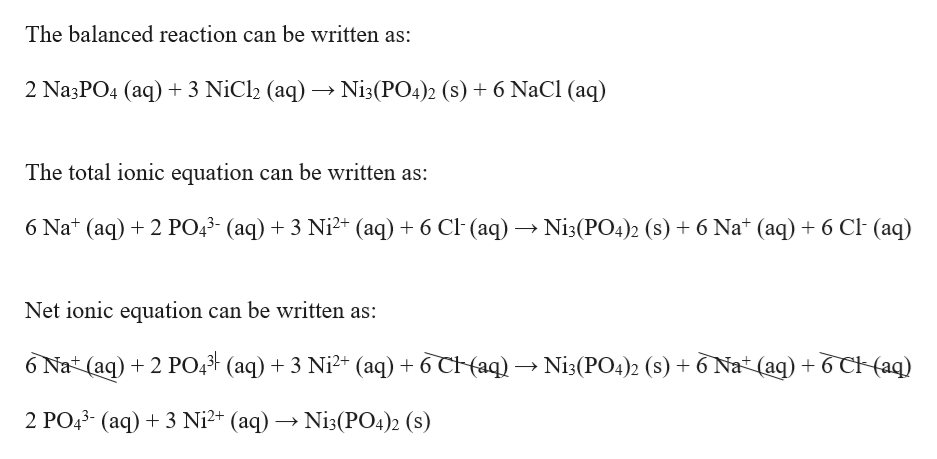Question

Write balanced net ionic equation for Na3PO4(aq)+NiCl2(aq)→Ni3(PO4)2(s)+NaCl(aq).

check_circleExpert Solution
Step 1

A balanced chemical equation or reaction is the one in which the total number of atoms for each element in the reactant side (left hand side) is equal to the total number of atoms for each element in the product side (right hand side).

The net ionic equation shows only the chemical species that are involved in a reaction, while the complete or total ionic equation&nbs...help_outlineImage TranscriptioncloseThe balanced reaction can be written as: Ni3(PO4)2 (s) + 6 NaCl (aq) 2 Na3PO4 (aq)3 NiCl2 (aq) The total ionic equation can be written as: 6 Nat (aq)2PO43- (aq)3 Ni2+ (aq) 6 Cl (aq) » Ni3(PO4)2 (s) 6 Nat (aq) 6 Cl (aq) Net ionic equation can be written as: 6 Nat (aq)2 PO43 (aq) 3 Ni2+ (aq)6 C (aq) » Ni3(PO4)2 (s) +6 Nat (aq) 6 CHaq) 2 PO43- (aq)3 Ni2+ (aq) -» Ni3(PO4)2 (s) fullscreen

### Want to see the full answer?

See Solution

#### Want to see this answer and more?

Solutions are written by subject experts who are available 24/7. Questions are typically answered within 1 hour*

See Solution
*Response times may vary by subject and question
Tagged in

### Chemistry